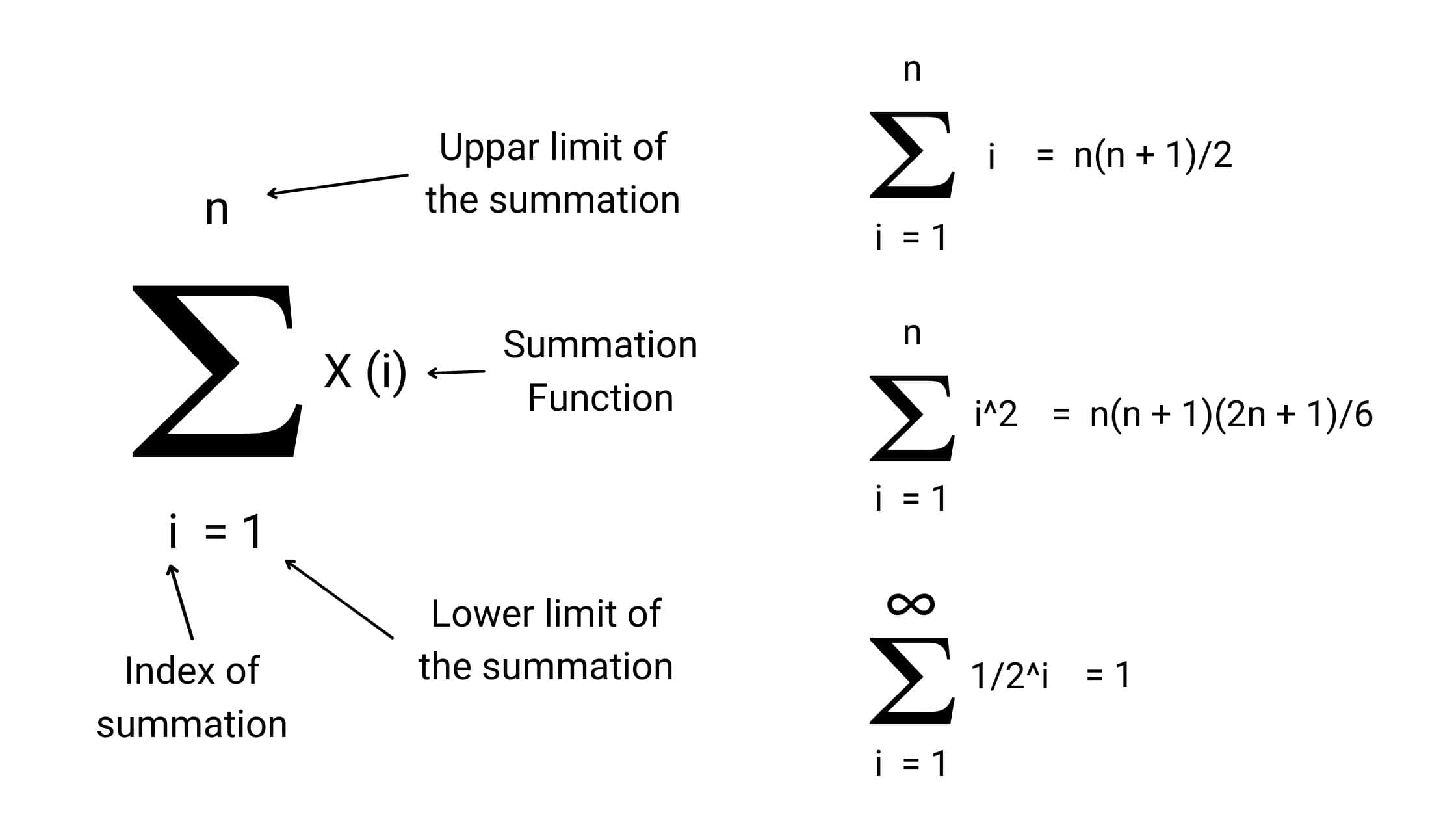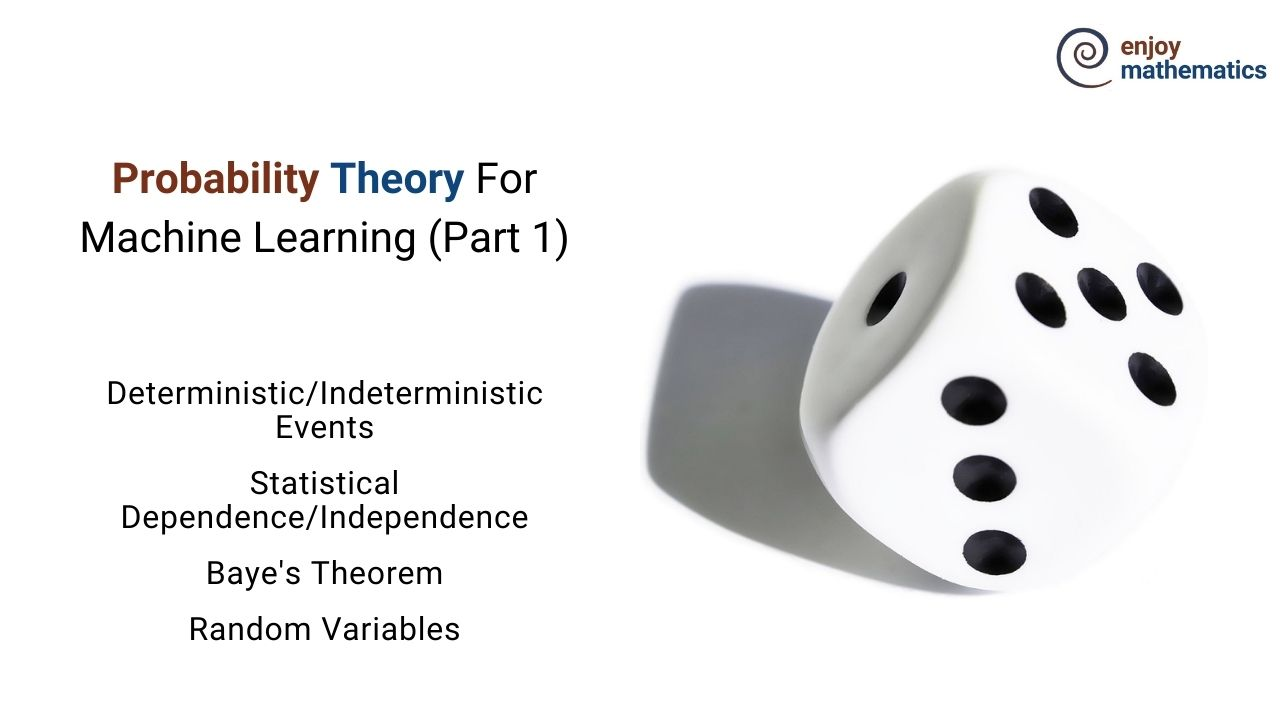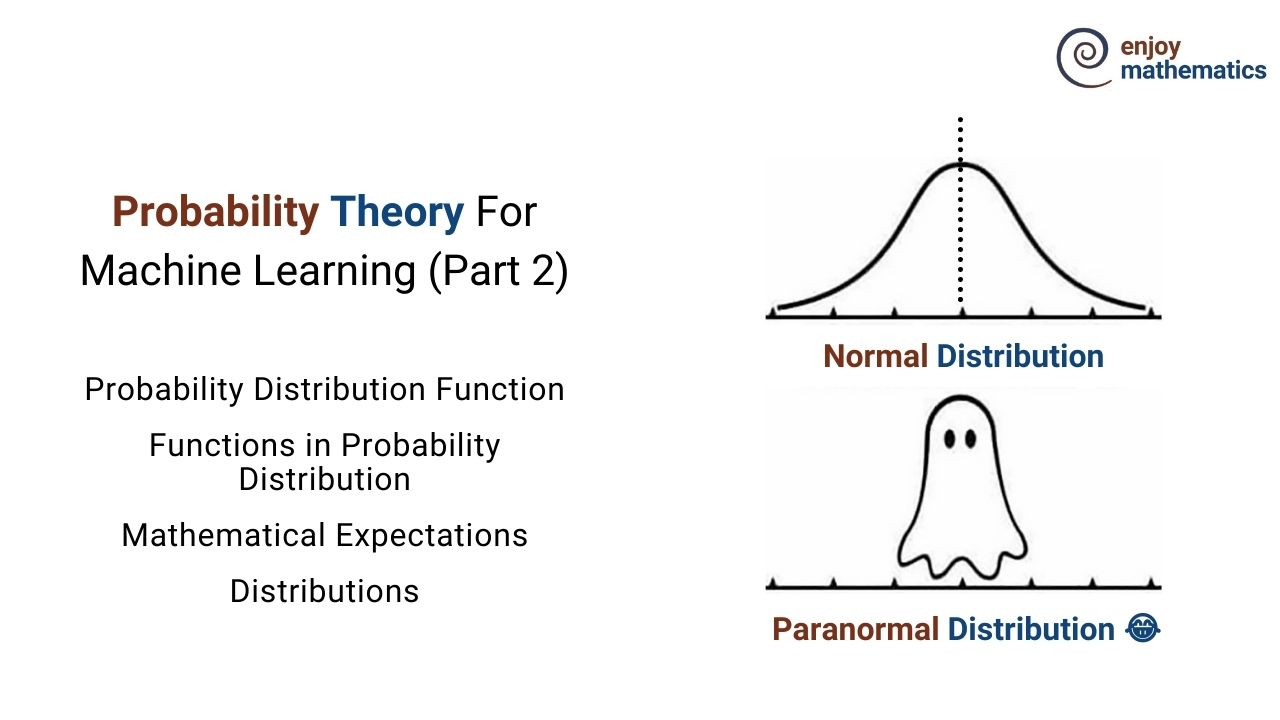# Enjoy Algorithmic Thinking

An initiative by enjoyalgorithms! We design content with in-depth explanations and help learners develop a dedicated interest in math, logic and algorithms.Important Math Topics for Data Structures and Algorithms

Just like programming, math is one of the core parts of learning data structures and algorithms. We mainly use math to analyse efficiency of various algorithms. But sometimes, the problem itself contains mathematical property or requires some mathematical insight to find a solution.Visual Proof: The Sum of Important Mathematical Series

The summation formulas are used to calculate the sum of the sequence. In this blog, we have discussed the visual proofs: the sum of numbers from 1 to n (arithmetic series), the sum of infinite geometric series, the sum of squares of numbers from 1 to n, etc.Probability Theory For Machine Learning (Part 1)

Originated from the “Games of Chance,” probability in itself is a branch of mathematics concerned about how likely it is that a proposition is true.Probability Distribution Function For Machine Learning

The Probability distribution lists the probabilities of the events that happened in any given random experiment. For example rolling a dice.

© 2020 Code Algorithms Pvt. Ltd.